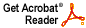# Notes on Oscillatory Integrals

Joel H. Shapiro

Lecture Notes 2012

Abstract: How does one get reasonable estimates of highly oscillatory definite integrals of functions like exp(itf(x)),  where f(x) = sin(x), x^2, ... , as t --> ∞? Why would one want to? These notes try to explain.

Download .pdf file (791 KB)